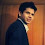# Joining and Merging in R

This tutorial explains how we can join (merge) two tables in R.

Let's create two tables -

Table I : DF1
df1 <- data.frame(ID = c(1, 2, 3, 4, 5),
w = c('a', 'b', 'c', 'd', 'e'),
x = c(1, 1, 0, 0, 1),
y=rnorm(5),
z=letters[1:5])

ID w x y z
1 a 1 -1.250974459 a
2 b 1 1.234389053 b
3 c 0 0.796469701 c
4 d 0 -0.004735964 d
5 e 1 -0.729994828 e

Table II : DF2
df2 <- data.frame(ID = c(1, 7, 3, 6, 8),
a = c('z', 'b', 'k', 'd', 'l'),
b = c(1, 2, 3, 0, 4),
c =rnorm(5),
d =letters[2:6])
ID a b c d
1 z 1 0.9367346 b
7 b 2 -2.3464766 c
3 k 3 0.8259913 d
6 d 0 -0.8663029 e
8 l 4 -0.482444 f
Inner Join
df3 = merge(df1, df2, by ="ID")
If the primary key (matching variable) do not have same name in both the tables (data frames),
df3 = merge(df1, df2, by.x ="ID", by.y="ID")
Left Join
df4 = merge(df1, df2, by ="ID", all.x = TRUE)
Right Join
df5 = merge(df1, df2, by ="ID", all.y = TRUE)
Full (Outer) Join
df6 = merge(df1, df2, by ="ID", all = TRUE)
Cross Join
df7 = merge(df1, df2, by = NULL)

With SQL Joins
library(sqldf)
df9 = sqldf('select df1.*, df2.* from df1 left join df2 on df1.ID = df2.ID')
Related Posts
ShareDeepanshu founded ListenData with a simple objective - Make analytics easy to understand and follow. He has over 10 years of experience in data science. During his tenure, he worked with global clients in various domains like Banking, Insurance, Private Equity, Telecom and HR.

3 Responses to "Joining and Merging in R"
1.Thank you for this

2.I have two files having different number of rows and column. I am trying to apply merge function because i was applying vlookup in excel. Still I am not getting the desired result in merge function which I was getting in vlookup function in excel. Please suggest me the solution. Also find the code which I am trying to run:

file1\$colname<-merge(file1,file2, by = "Symbol")

1.Try this :

file1\$colname<-merge(file1,file2, by = "Symbol", all.x = TRUE)

Next → ← Prev
Looks like you are using an ad blocker!

To continue reading you need to turnoff adblocker and refresh the page. We rely on advertising to help fund our site. Please whitelist us if you enjoy our content.